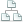Home >Tips >Calculate Costs With ExcelSite map

Analyze costs with Excel 2010

An Excel tutorial by Peter Kalmstrom

Here the kalmstrom.com CEO Peter Kalmstrom shows how to calculate costs with Excel 2010. As example Peter has chosen to analyze his own costs for a holiday trip, but the Excel model may of course be used for any cost calculation.

In the process Peter shows many things that you might find useful also when you work with other tasks in Excel. These are among the tips and tricks Peter shares in the demo:
• copy formatting
• copy currency
• give names to cells to use them in formulas
• format text as tables